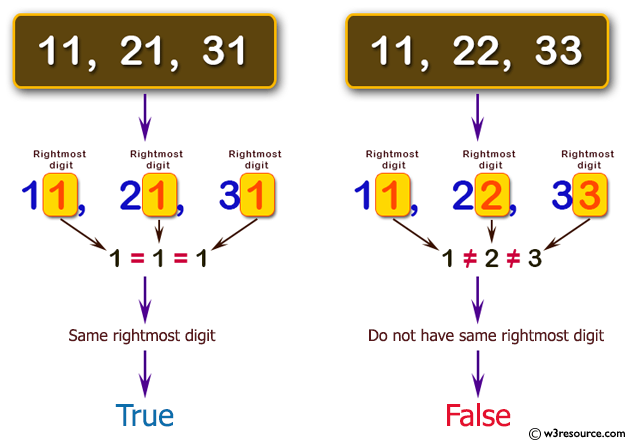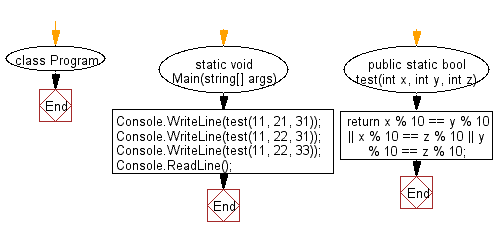﻿ C# - An integer with two or more rightmost digits# C# Sharp Basic Algorithm Exercises: Check whether two or more non-negative given integers have the same rightmost digit

## C# Sharp Basic Algorithm: Exercise-50 with Solution

Write a C# Sharp program to check whether two or more non-negative given integers have the same rightmost digit.

Pictorial Presentation:Sample Solution:-

C# Sharp Code:

``````using System;
using System.Linq;
namespace exercises
{
class Program
{
static void Main(string[] args)
{
Console.WriteLine(test(11, 21, 31));
Console.WriteLine(test(11, 22, 31));
Console.WriteLine(test(11, 22, 33));
}
public static bool test(int x, int y, int z)
{
return x % 10 == y % 10 || x % 10 == z % 10 || y % 10 == z % 10;
}
}
}
```
```

Sample Output:

```True
True
False```

Flowchart:C# Sharp Code Editor:

Improve this sample solution and post your code through Disqus

What is the difficulty level of this exercise?

Test your Programming skills with w3resource's quiz.

﻿